# 3-D Speech Enhancement Using Trained Filter and Sum Network

In this example, you perform speech enhancement using a pretrained deep learning model. For details about the model and how it was trained, see Train 3-D Speech Enhancement Network Using Deep Learning (Audio Toolbox). The speech enhancement model is an end-to-end deep beamformer that takes B-format ambisonic audio recordings and outputs enhanced mono speech signals.

Download the pretrained speech enhancement (SE) network, ambisonic test files, and labels. The model architecture is based on  and , as implemented in the baseline system for the L3DAS21 challenge task 1 . The data the model was trained on and the ambisonic test files are provided as part of .

```downloadFolder = matlab.internal.examples.downloadSupportFile("audio","speechEnhancement/FaSNet.zip"); dataFolder = tempdir; unzip(downloadFolder,dataFolder) netFolder = fullfile(dataFolder,"speechEnhancement"); addpath(netFolder)```

Load the clean speech and listen to it.

```[cleanSpeech,fs] = audioread("cleanSpeech.wav"); soundsc(cleanSpeech,fs)```

In the L3DAS21 challenge, "clean" speech files were taken from the LibriSpeech dataset and augmented to obtain synthetic tridimensional acoustic scenes containing a randomly placed speaker and other sound sources typical of background noise in an office environment. The data is encoded as B-format ambisonics. Load the ambisonic data. First order B-format ambisonic channels correspond to the sound pressure captured by an omnidirectional microphone (W) and sound pressure gradients X, Y, and Z that correspond to front/back, left/right, and up/down captured by figure-of-eight capsules oriented along the three spatial axes.

`[ambisonicData,fs] = audioread("ambisonicRecording.wav");`

Listen to a channel of the ambisonic data.

```channel =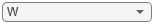1; soundsc(ambisonicData(:,channel),fs)```

To plot the clean speech and the noisy ambisonic data, use the supporting function `compareAudio`.

`compareAudio(cleanSpeech,ambisonicData,SampleRate=fs)`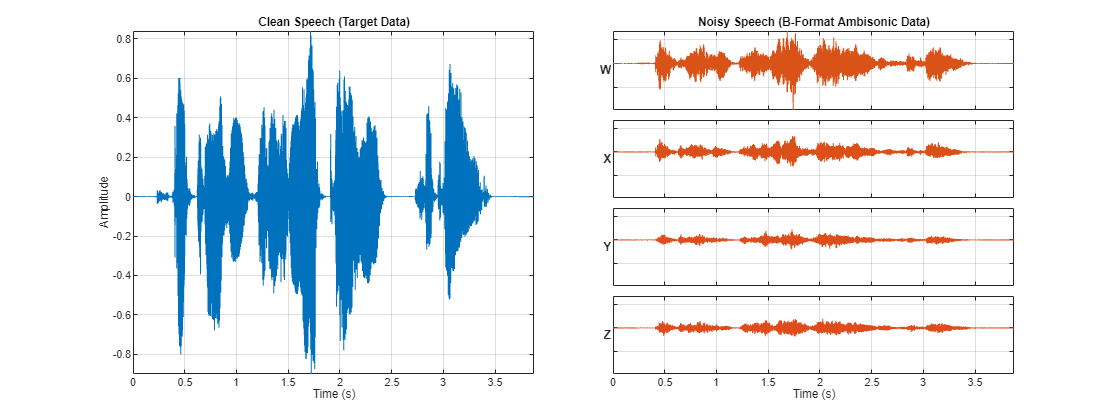To visualize the spectrograms of the clean speech and the noisy ambisonic data, use the supporting function `compareSpectrograms`.

`compareSpectrograms(cleanSpeech,ambisonicData)`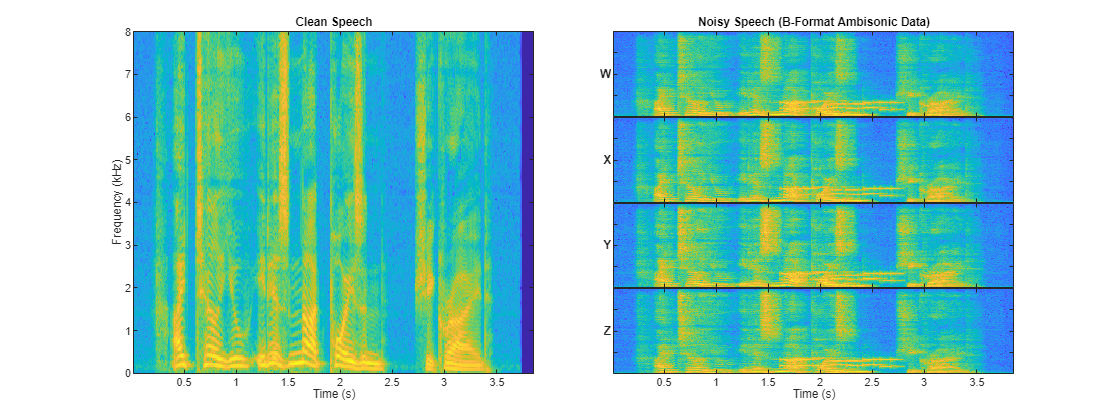Mel spectrograms are auditory-inspired transformations of spectrograms that emphasize, de-emphasize, and blur frequencies similar to how the auditory system does. To visualize the mel spectrograms of the clean speech and the noisy ambisonic data, use the supporting function `compareSpectrograms` and set `Warp` to `mel`.

`compareSpectrograms(cleanSpeech,ambisonicData,Warp="mel")`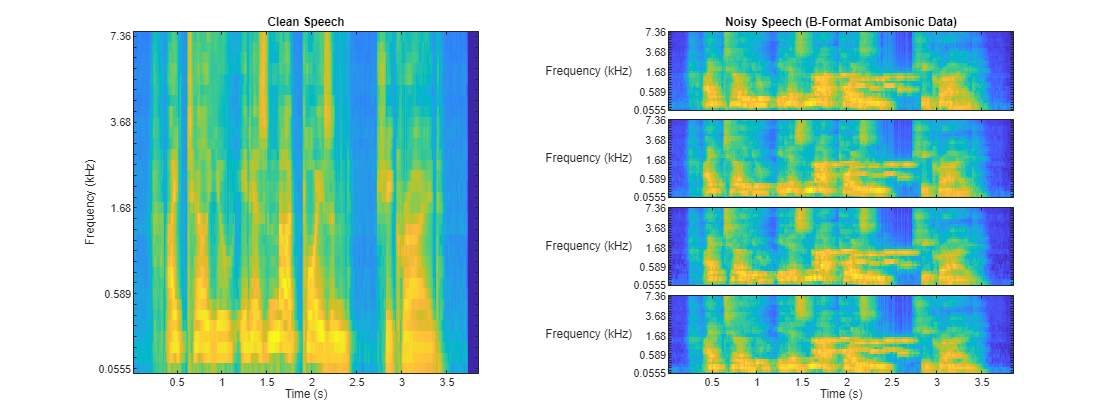### Perform 3-D Speech Enhancement

Use the supporting object, `seModel`, to perform speech enhancement. The `seModel` class definition is in the current folder when you open this example. The object encapsulates the SE model developed in Train 3-D Speech Enhancement Network Using Deep Learning (Audio Toolbox). Create the model, then call `enhanceSpeech` on the ambisonic data to perform speech enhancement.

```model = seModel(netFolder); enhancedSpeech = enhanceSpeech(model,ambisonicData);```

Listen to the enhanced speech. You can compare the enhanced speech listening experience with the clean speech or noisy ambisonic data by selecting the desired sound source from the dropdown.

```soundSource =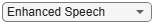enhancedSpeech; soundsc(soundSource,fs)```

Compare the clean speech, noisy speech, and enhanced speech in the time domain, as spectrograms, and as mel spectrograms.

`compareAudio(cleanSpeech,ambisonicData,enhancedSpeech)`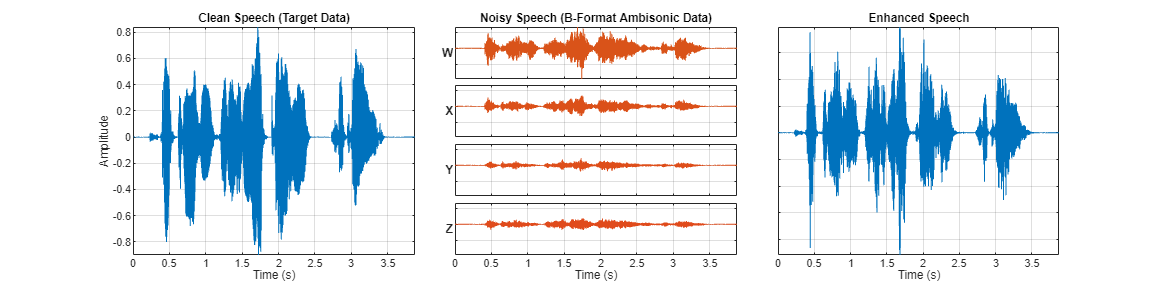`compareSpectrograms(cleanSpeech,ambisonicData,enhancedSpeech)`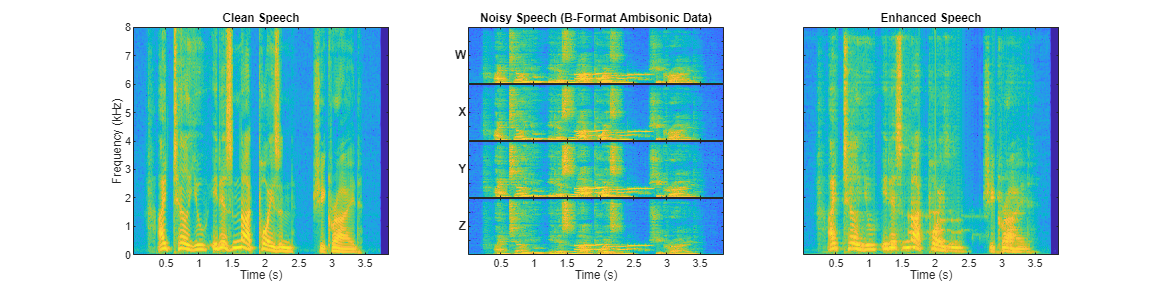`compareSpectrograms(cleanSpeech,ambisonicData,enhancedSpeech,Warp="mel")`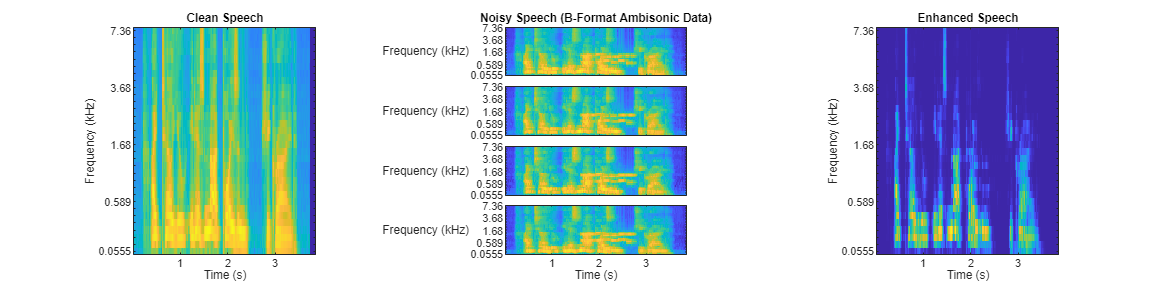### Speech Enhancement for Speech-to-Text Applications

Compare the performance of the speech enhancement system on a downstream speech-to-text system. Use the wav2vec 2.0 speech-to-text model. This model requires a one-time download of pretrained weights to run. If you have not downloaded the wav2vec weights, the first call to `speechClient` will provide a download link.

Create the wav2vec 2.0 speech client to perform transcription.

`transcriber = speechClient("wav2vec2.0",segmentation="none");`

Perform speech-to-text transcription using the clean speech, the ambisonic data, and the enhanced speech.

`cleanSpeechResults = speech2text(transcriber,cleanSpeech,fs)`
```cleanSpeechResults = "i tell you it is not poison she cried" ```
`noisySpeechResults = speech2text(transcriber,ambisonicData(:,channel),fs)`
```noisySpeechResults = "i tell you it is not parzona she cried" ```
`enhancedSpeechResults = speech2text(transcriber,enhancedSpeech,fs)`
```enhancedSpeechResults = "i tell you it is not poison she cried" ```

### Speech Enhancement for Telecommunications Applications

Compare the performance of the speech enhancement system using the short-time objective intelligibility (STOI) measurement . STOI has been shown to have a high corelation with the intelligibility of noisy speech and is commonly used to evaluate speech enhancement systems.

Calculate STOI for the omnidirectional channel of the ambisonics, and for the enhanced speech. Perfect intelligibility has a score of 1.

`stoi(cleanSpeech,ambisonicData(:,channel),fs)`
```ans = 0.6950 ```
`stoi(cleanSpeech,enhancedSpeech,fs)`
```ans = 0.8393 ```

### References

 Luo, Yi, Cong Han, Nima Mesgarani, Enea Ceolini, and Shih-Chii Liu. "FaSNet: Low-Latency Adaptive Beamforming for Multi-Microphone Audio Processing." In 2019 IEEE Automatic Speech Recognition and Understanding Workshop (ASRU), 260–67. SG, Singapore: IEEE, 2019. https://doi.org/10.1109/ASRU46091.2019.9003849.

 Guizzo, Eric, Riccardo F. Gramaccioni, Saeid Jamili, Christian Marinoni, Edoardo Massaro, Claudia Medaglia, Giuseppe Nachira, et al. "L3DAS21 Challenge: Machine Learning for 3D Audio Signal Processing." In 2021 IEEE 31st International Workshop on Machine Learning for Signal Processing (MLSP), 1–6. Gold Coast, Australia: IEEE, 2021. https://doi.org/10.1109/MLSP52302.2021.9596248.

 Roux, Jonathan Le, et al. "SDR – Half-Baked or Well Done?" ICASSP 2019 - 2019 IEEE International Conference on Acoustics, Speech and Signal Processing (ICASSP), IEEE, 2019, pp. 626–30. DOI.org (Crossref), https://doi.org/10.1109/ICASSP.2019.8683855.

 Luo, Yi, et al. "Dual-Path RNN: Efficient Long Sequence Modeling for Time-Domain Single-Channel Speech Separation." ICASSP 2020 - 2020 IEEE International Conference on Acoustics, Speech and Signal Processing (ICASSP), IEEE, 2020, pp. 46–50. DOI.org (Crossref), https://doi.org/10.1109/ICASSP40776.2020.9054266.

 Taal, Cees H., Richard C. Hendriks, Richard Heusdens, and Jesper Jensen. "An Algorithm for Intelligibility Prediction of Time–Frequency Weighted Noisy Speech." IEEE Transactions on Audio, Speech, and Language Processing 19, no. 7 (September 2011): 2125–36. https://doi.org/10.1109/TASL.2011.2114881.

### Supporting Functions

#### Compare Audio

```function compareAudio(target,x,y,parameters) %compareAudio Plot clean speech, B-format ambisonics, and predicted speech % over time arguments target x y = [] parameters.SampleRate = 16e3 end numToPlot = 2 + ~isempty(y); f = figure; tiledlayout(4,numToPlot,TileSpacing="compact",TileIndexing="columnmajor") f.Position = [f.Position(1),f.Position(2),f.Position(3)*numToPlot,f.Position(4)]; t = (0:(size(x,1)-1))/parameters.SampleRate; xmax = max(x(:)); xmin = min(x(:)); nexttile(1,[4,1]) plot(t,target,Color=[0 0.4470 0.7410]) axis tight ylabel("Amplitude") xlabel("Time (s)") title("Clean Speech (Target Data)") grid on nexttile(5) plot(t,x(:,1),Color=[0.8500 0.3250 0.0980]) title("Noisy Speech (B-Format Ambisonic Data)") axis([t(1),t(end),xmin,xmax]) set(gca,Xticklabel=[],YtickLabel=[]) grid on yL = ylabel("W",FontWeight="bold"); set(yL,Rotation=0,VerticalAlignment="middle",HorizontalAlignment="right") nexttile(6) plot(t,x(:,2),Color=[0.8600 0.3150 0.0990]) axis([t(1),t(end),xmin,xmax]) set(gca,Xticklabel=[],YtickLabel=[]) grid on yL = ylabel("X",FontWeight="bold"); set(yL,Rotation=0,VerticalAlignment="middle",HorizontalAlignment="right") nexttile(7) plot(t,x(:,3),Color=[0.8700 0.3050 0.1000]) axis([t(1),t(end),xmin,xmax]) set(gca,Xticklabel=[],YtickLabel=[]) grid on yL = ylabel("Y",FontWeight="bold"); set(yL,Rotation=0,VerticalAlignment="middle",HorizontalAlignment="right") nexttile(8) plot(t,x(:,4),Color=[0.8800 0.2950 0.1100]) axis([t(1),t(end),xmin,xmax]) xlabel("Time (s)") set(gca,YtickLabel=[]) grid on yL = ylabel("Z",FontWeight="bold"); set(yL,Rotation=0,VerticalAlignment="middle",HorizontalAlignment="right") if numToPlot==3 nexttile(9,[4,1]) plot(t,y,Color=[0 0.4470 0.7410]) axis tight xlabel("Time (s)") title("Enhanced Speech") grid on set(gca,YtickLabel=[]) end end```

#### Compare Spectrograms

```function compareSpectrograms(target,x,y,parameters) %compareSpectrograms Plot spectrograms of clean speech, B-format % ambisonics, and predicted speech over time arguments target x y = [] parameters.SampleRate = 16e3 parameters.Warp = "linear" end fs = parameters.SampleRate; switch parameters.Warp case "linear" fn = @(x)spectrogram(x,hann(round(0.03*fs),"periodic"),round(0.02*fs),round(0.03*fs),fs,"onesided","power","yaxis"); case "mel" fn = @(x)melSpectrogram(x,fs); end numToPlot = 2 + ~isempty(y); f = figure; tiledlayout(4,numToPlot,TileSpacing="tight",TileIndexing="columnmajor") f.Position = [f.Position(1),f.Position(2),f.Position(3)*numToPlot,f.Position(4)]; nexttile(1,[4,1]) fn(target) fh = gcf; fh.Children(1).Children(1).Visible="off"; title("Clean Speech") nexttile(5) fn(x(:,1)) fh = gcf; fh.Children(1).Children(1).Visible="off"; set(gca,Yticklabel=[],XtickLabel=[],Xlabel=[]) yL = ylabel("W",FontWeight="bold"); set(yL,Rotation=0,VerticalAlignment="middle",HorizontalAlignment="right") title("Noisy Speech (B-Format Ambisonic Data)") nexttile(6) fn(x(:,2)) fh = gcf; fh.Children(1).Children(1).Visible="off"; set(gca,Yticklabel=[],XtickLabel=[],Xlabel=[]) yL = ylabel("X",FontWeight="bold"); set(yL,Rotation=0,VerticalAlignment="middle",HorizontalAlignment="right") nexttile(7) fn(x(:,3)) fh = gcf; fh.Children(1).Children(1).Visible="off"; set(gca,Yticklabel=[],XtickLabel=[],Xlabel=[]) yL = ylabel("Y",FontWeight="bold"); set(yL,Rotation=0,VerticalAlignment="middle",HorizontalAlignment="right") nexttile(8) fn(x(:,4)) fh = gcf; fh.Children(1).Children(1).Visible="off"; set(gca,Yticklabel=[]) yL = ylabel("Z",FontWeight="bold"); set(yL,Rotation=0,VerticalAlignment="middle",HorizontalAlignment="right") if numToPlot==3 nexttile(9,[4,1]) fn(y) fh = gcf; fh.Children(1).Children(1).Visible="off"; set(gca,Yticklabel=[],Ylabel=[]) title("Enhanced Speech") end end```

#### Short-Time Objective Intelligibility (STOI) Measure

```function metric = stoi(t,y,fs) %STOI Short-time objective intelligibility measure (STOI) % metric = stoid(t,y,fs) returns the short-time objective intelligibility % measurement (STOI) defined in  and . t is the clean speech signal, % y is the predicted speech signal, and fs is the sample rate. % % References %  C. H. Taal, R. C. Hendriks, R. Heusdens, and J. Jensen. A Short-Time % Objective Intelligibility Measure for Time-Frequency Weighted Noisy % Speech. In Acoustics Speech and Signal Processing (ICASSP), pages % 4214-4217. IEEE, 2010. % %  C. H. Taal, R. C. Hendriks, R. Heusdens, and J. Jensen. An Algorithm % for Intelligibility Prediction of Time-Frequency Weighted Noisy Speech. % IEEE Transactions on Audio, Speech and Language Processing, % 19(7):2125-2136, 2011. % Define parameters designFs = 10e3; windowLength = 256; fttLength = 512; numBands = 15; cf1 = 150; % Center frequency of first 1/3 octave band in Hz. N = 30; % Number of frames for intermediate intelligibility Beta = -15; % Lower bound of signal to distortion ratio (SDR) clipConstant = 10^(-Beta/20); energyThreshold = 40; % Speech dynamic range if fs ~= designFs t = cast(resample(double(t),designFs,fs),like=t); y = cast(resample(double(y),designFs,fs),like=y); end % Remove silent frames [t,y] = removeSilentFrames(t,y,energyThreshold,windowLength); % Compute magnitude short-time Fourier transform T = stft(t,FFTLength=fttLength,Window=hann(windowLength), ... OverlapLength=windowLength/2,FrequencyRange="onesided"); Y = stft(y,FFTLength=fttLength,Window=hann(windowLength), ... OverlapLength=windowLength/2,FrequencyRange="onesided"); T = abs(T); Y = abs(Y); % Design frequency-domain octave filter bank fb = designOctaveFilterBank(designFs,fttLength,numBands,cf1); % Apply octave filter bank T = fb*T; Y = fb*Y; % Compute intelligibility measurement djm = zeros(numBands,length(N:size(T,2))); for m = N:size(T,2) % Isolate region of N consecutive TF-units Tj = T(:,(m-N+1):m); Yj = Y(:,(m-N+1):m); % Calculate alpha alpha = sqrt(sum(Tj.^2,2)./sum(Yj.^2,2)); alphaYj = Yj.*alpha; Ypj = min(alphaYj,Tj+Tj*clipConstant); % Eq 3 from  % Eq 4 from  yn = Ypj - mean(Ypj,2); xn = Tj - mean(Tj,2); djm(:,m-N+1) = dot(xn./vecnorm(xn,2,2),yn./vecnorm(yn,2,2),2); end % Average intermediate intelligibility over all bands and frames (eq 5 in % ) metric = mean(djm(:)); % Remove Silent Frames function [tS,yS] = removeSilentFrames(t,y,eThreshold,N) win = hanning(N); tb = buffer(t,N,N/2,"nodelay"); tbwin = tb.*win; frameEnergy = 20*log10(vecnorm(tbwin)./sqrt(N)); mask = (frameEnergy-max(frameEnergy)+eThreshold)>0; tS = tbwin; tS(:,~mask) = []; tS = tS(1:N/2,2:end) + tS(N/2+1:end,1:end-1); tS = [tb(1:N/2,1);tS(:);tb(N/2+1:end,end)]; yb = buffer(y,N,N/2,"nodelay"); ybwin = yb.*win; yS = ybwin; yS(:,~mask) = []; yS = yS(1:N/2,2:end) + yS(N/2+1:end,1:end-1); yS = [yb(1:N/2,1);yS(:);yb(N/2+1:end,end)]; end % Design Octave Filter Bank function fb = designOctaveFilterBank(fs,fftLength,numBands,cf1) f = linspace(0,fs,fftLength+1); f = f(1:(fftLength/2+1)); k = 0:(numBands-1); cf = 2.^(k/3)*cf1; fl = sqrt((2.^(k/3)*cf1).*2.^((k-1)/3)*cf1); fr = sqrt((2.^(k/3)*cf1).*2.^((k+1)/3)*cf1); fb = zeros(numBands,numel(f)); fp = f'; [~,bandLow] = min((fp-fl).^2); [~,bandHigh] = min((fp-fr).^2); for ii = 1:numel(cf) fb(ii,bandLow(ii):(bandHigh(ii)-1)) = 1; end end end```